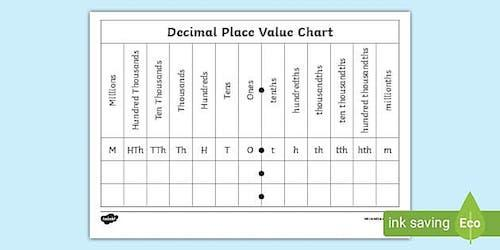# Understanding The Decimal Place Value

As you probably already know, a decimal number is defined as a number that is written in decimal notation where each digit represents the different powers of 10. For example, 20.456, 5.^006, 0.0001 are all examples of decimal numbers.

Check out all our rounding calculators.

We can then state that a decimal number consists of the following parts:

## #1: The Whole Number Part:

For example, 5 is the whole number in 5.006.

## #2: The Decimal Part:

For example, 456 is the decimal part in 20.456.

Notice that the whole and decimal parts are separated by a dot (.) that is known as the decimal point. So, simply put, the decimal point separates the whole number part on the left side and decimal part or fractional part on the right part.

The places on the left side or whole number part begin with ones, followed by tens, then hundreds, followed by thousands, and so forth. On the other hand, the places on the right or fractional part begin with tenths, followed by hundredths, then thousandths, and so forth.

Learn how to round decimals to the nearest whole number.

## How To Read Decimals?

In case you are wondering about how to read decimals, you need to know that you have two different ways to do it.

## #1: Reading Off The Digits Of A Decimal Number:

For example:

• 0.005 is read as “zero point zero, zero, five”
• 4.2 is read as “four, point two”
• 578 is written and read as twenty-five, point five seven eight
• 14 is written and read as seven thousand, point one four
• 002 is written and read as zero point zero, zero two

Check out this rounding decimals worksheet.

## #2: Reading The Decimal Part Number As If It Was A Single Integer:

For example:

• 42.37 is read as forty-two and thirty-seven hundredths
• 8.34 is read as eight and thirty-four hundredths.
• 0.578 is written and read as twenty-five and five hundred seventy-eight thousandths.
• 0.14 is written and read as seven thousand and fourteen hundredths.
• 0.002 is written and read as two thousandths.

## What Is The Decimal Place Value?

Simply put, the decimal place value is a positional notation system where the position of an integer in a number and with respect to a point, determines the value of it. The decimal place value is done exactly the same way whole numbers are treated, but in this case, it is reverse.

The place value in decimals is based on the preceding exponential of 10. When shifting from the left to the right of a decimal number, the integers get divided by powers of 10 (10^-1= 1/10, 10^-2 =1/100, 10^-3= 1/1000, etc.), implying that, the decimal place value defines the tenths, hundredths, and so on. A hundredth in a decimal number implies 1/100 or 0.01.

Looking to learn how to divide decimals by a decimal?

Here is a simple example:

Determine the place of the decimal digits in the following number: 0.37.

Solution

The number 3 is in the place of tenths, and so the place value is:

3 x 10^-1 = 3/10 = 0.3

7 is in the position of hundredths, and hence its place value is:

7 x 10^-2 = 7/100 = 0.07

© 2022 rounding.to . Powered by WordPress. Theme by Viva Themes.